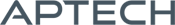# Optimization MT

Optimization MT

Availability: Out of stock

Optimization MT (OPTMT) provides a suite of tools for the unconstrained optimization of functions. It has many features, including a wide selection of descent algorithms, step-length methods, and "on-the-fly" algorithm switching.
Overview

## Optimization MT 2.0

OPMT is intended for the optimization of functions. It has many features, including a wide selection of descent algorithms, step-length methods, and "on-the-fly" algorithm switching. Default selections permit you to use Optimization with a minimum of programming effort. All you provide is the function to be optimized and start values, and OPMT does the rest.

## Version 2.0 is easier to use than ever!

• New syntax options eliminate the need for PV and DS structures:
• Decreasing the required code up to 25%.
• Decreasing runtime up to 20%.
• Simplifying usage.
• Optional dynamic arguments make it simple and transparent to add extra data arguments beyond model parameters to your objective function.
• Updated documentation and examples.
• Fully backwards compatible with OPTMT 1.0

Platform: Windows, Mac, and Linux.

Requirements: GAUSS/GAUSS Engine/GAUSS Light v16 or higher.

Features

## Key Features

### Descent methods

• BFGS (Broyden, Fletcher, Goldfarb and Powell)
• DFP (Davidon, Fletcher and Powell)
• Newton
• Steepest Descent

### Line search methods

• STEPBT
• Brent’s method
• HALF
• Strong Wolfe’s Conditions New!

### Flexible

• Bounded parameters.
• Specify fixed and free parameters.
• Dynamic algorithm switching.
• Compute all, a subset, or none of the derivatives numerically.
• Easily pass data other than the model parameters as extra input arguments. New!

### Efficient

• Option to avoid computations that are the same for the objective function and derivatives.
• The tremendous speed of user-defined procedures in GAUSS speeds up your optimization problems.

### Trusted

• For more than 30 years, leading researchers have trusted the efficient and numerically sound code in the GAUSS optimization packages to keep them at the forefront of their fields.

## Details

Novice users will typically leave most of these options at the default values. However, they can be a great help when tackling more difficult problems.

Control options
Parameter boundsSimple parameter bounds of the type: lower_bd ≤ x_i ≤ upper_bd
Descent algorithmsBFGS, DFP, Newton and Steepest Descent.
Algorithm switchingSpecify descent algorithms to switch between based upon the number of elapsed iterations, a minimum change in the objective function or line search step size.
Line search methodSTEPBT (quadratic and cubic curve fit), Brent’s method, half-step or Strong Wolfe’s Conditions.
Active parametersControl which parameters are active (to be estimated) and which should be fixed to their start value.
Gradient MethodEither compute an analytical gradient, or have OPTMT compute a numerical gradient using the forward, central or backwards difference method.
Hessian MethodEither compute an analytical Hessian, or have OPTMT compute a numerical Hessian using the forward, central or backwards difference method.
Random seedStarting seed value used by the random line search method to allow for repeatable code.
Print outputControls whether (or how often) iteration output is printed and whether a final report is printed.
Gradient stepAdvanced feature: Controls the increment size for computing the step size for numerical first and second derivatives.
Maximum iterationsMaximum iterations to converge.
Maximum elapsed timeMaximum number of minutes to converge.
Maximum random search attemptsMaximum allowed number of random line search attempts.
Convergence toleranceConvergence is achieved when the direction vector changes less than this amount.

Examples

The code below finds the minimum of the simple function x2. The objective function in this case computes the function value and/or the gradient, depending upon the value of the incoming indicator vector, ind. This makes it simple to avoid duplication of calculations which are required for the objective function and the gradient when computing more complicated functions.

``````//Load optmt library
library optmt;

//Objective function to be minimized
proc fct(x, ind);

//Declare 'mm' to be a modelResults
//struct, local to this procedure
struct modelResults mm;

//If the first element of the indicator vector
//is non-zero, calculate the objective function
if ind;
//Assign the value of the objective function to the
//'function' member of the 'modelResults' struct
mm.function = x.^2;
endif;

//If the second element of the indicator vector
if ind;
//Assign the value of the objective function to the
//'function' member of the 'modelResults' struct
endif;

//Return the modelResults structure
retp(mm);
endp;

// Starting parameter value
x0 = 1;

//Declare 'out' to be an optmtResults struct
//to hold the optimization results
struct optmtResults out;

//Minimize objective function
out = optmt(&fct,x0);

//Print optimtization results
optmtPrt(out);``````

The above code will print the simple report below. It shows that OPTMT has found the minimum of our function x2 when x is equal to 0. We also see that the function value is equal to 0 which we expect and no parameter bounds were active, because the Lagrangians are an empty matrix.

```=========================================
Optmt Version 2.0.1
=========================================

Return code    =    0
Function value =    0.00000
Convergence    :    normal convergence

----------------------------------------
x[1,1]      0.0000      0.0000

Number of iterations    2
Minutes to convergence     0.00000

Lagrangians
{}```

Product Inquiry

8 + 9 = enter the result ex:(3 + 2 = 5)

﻿